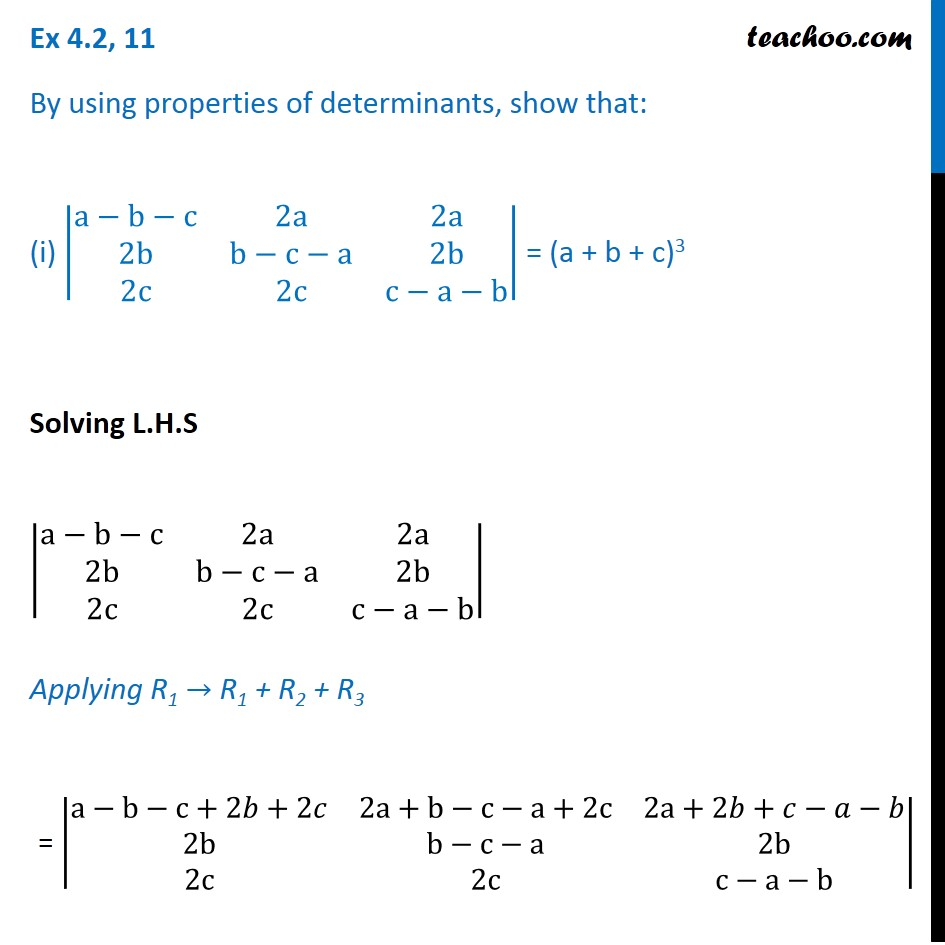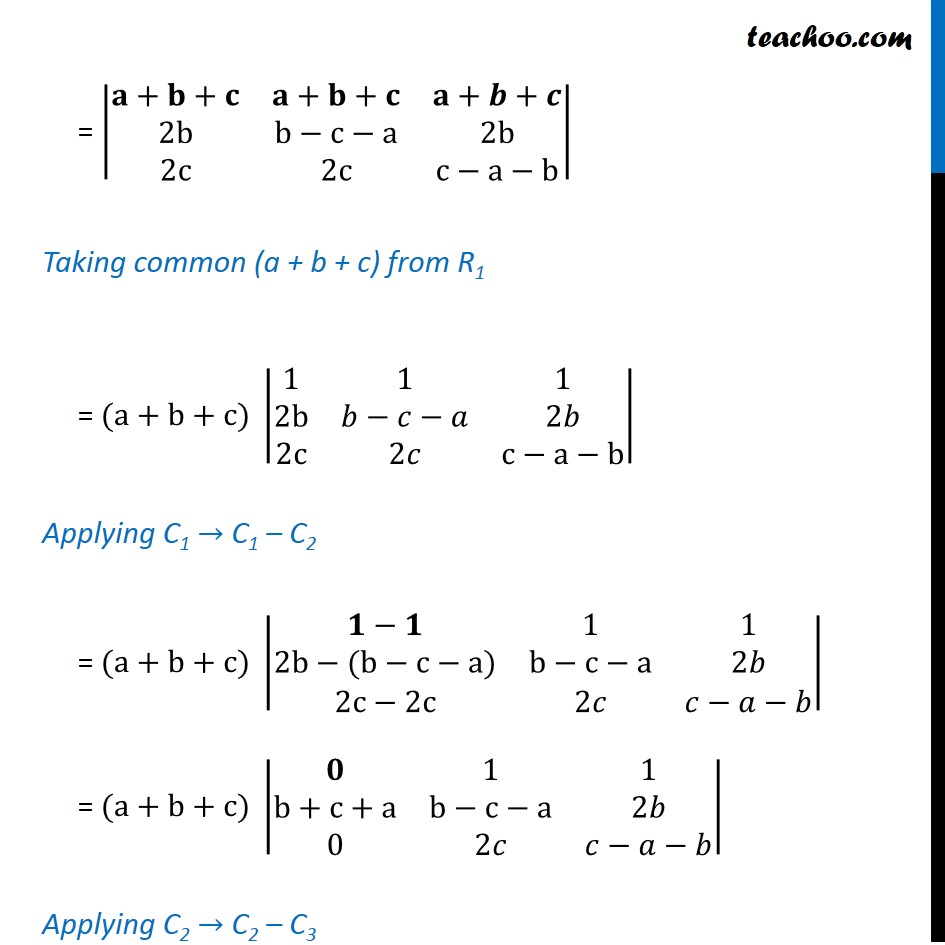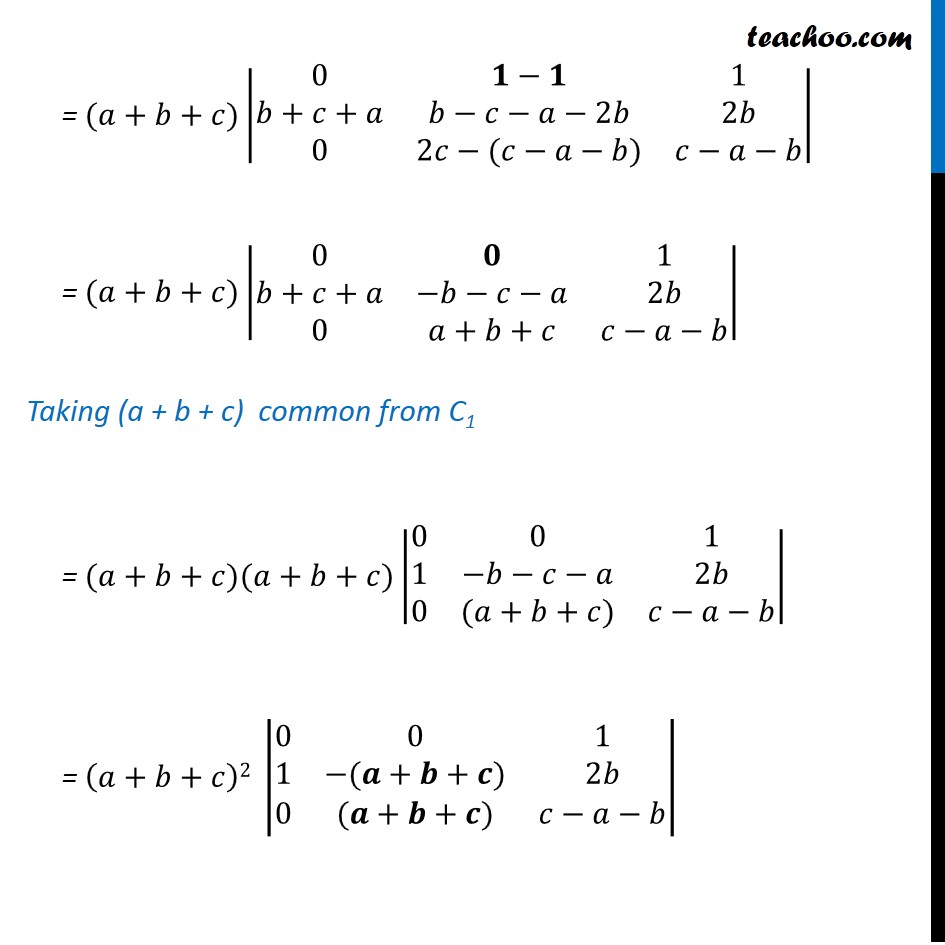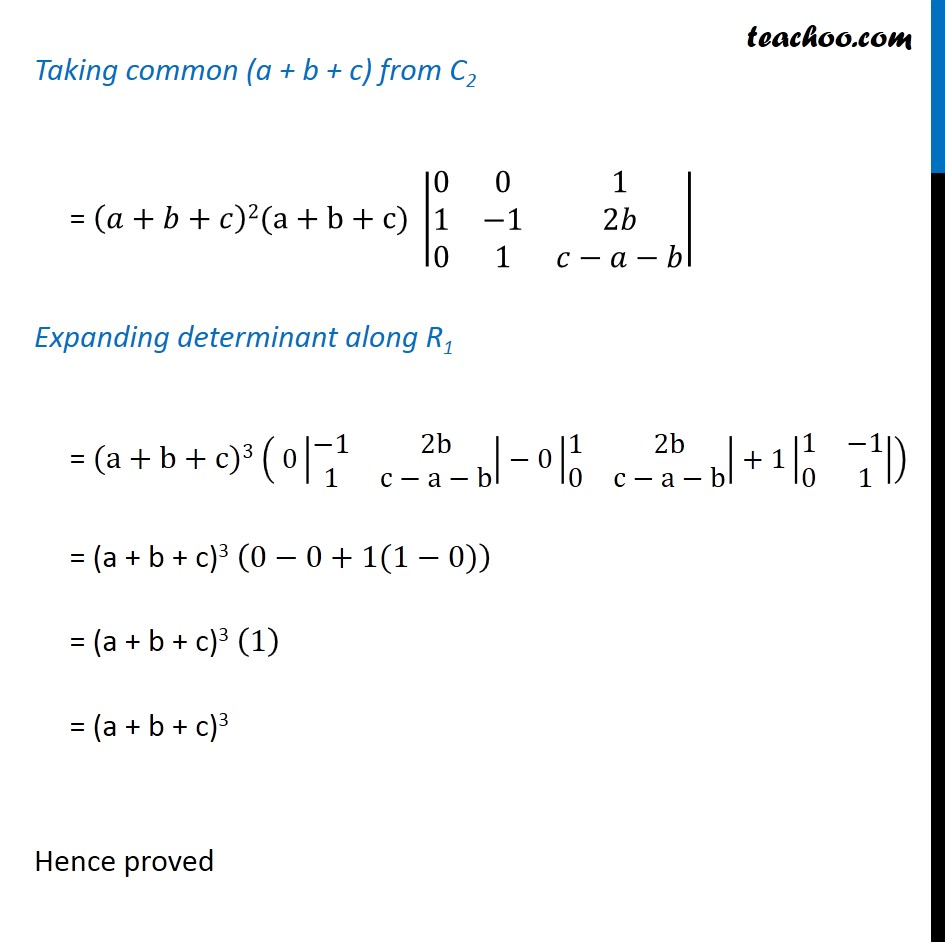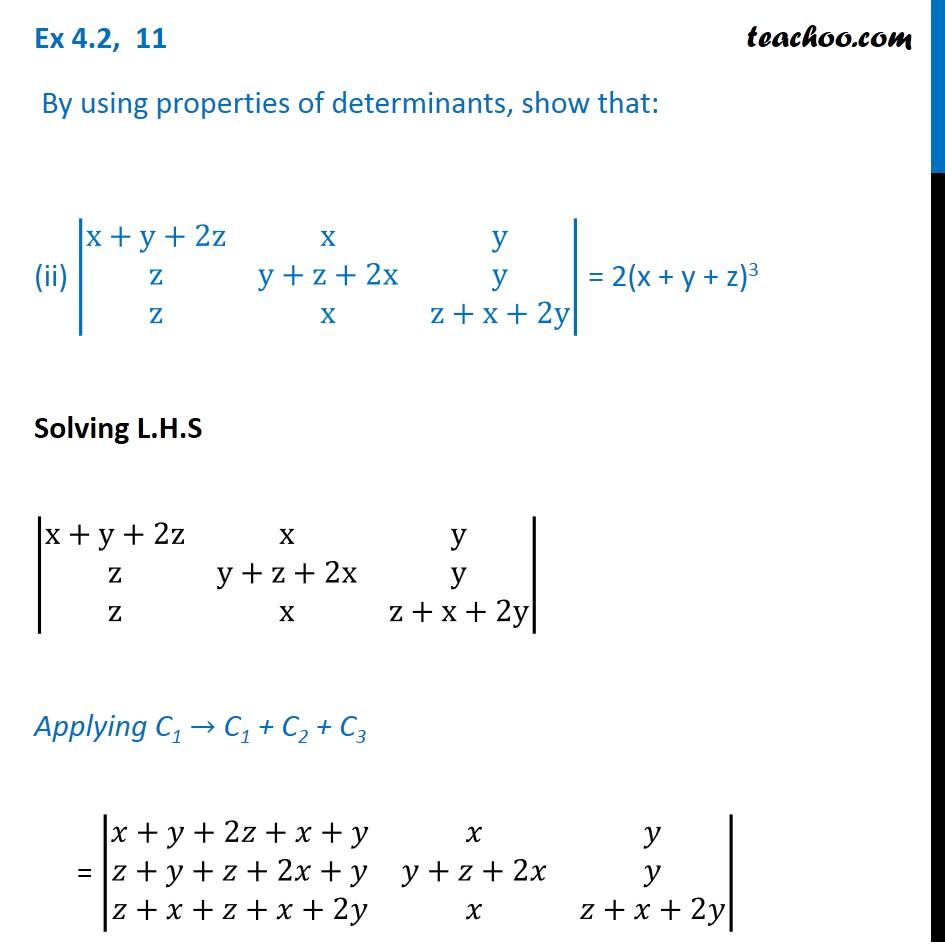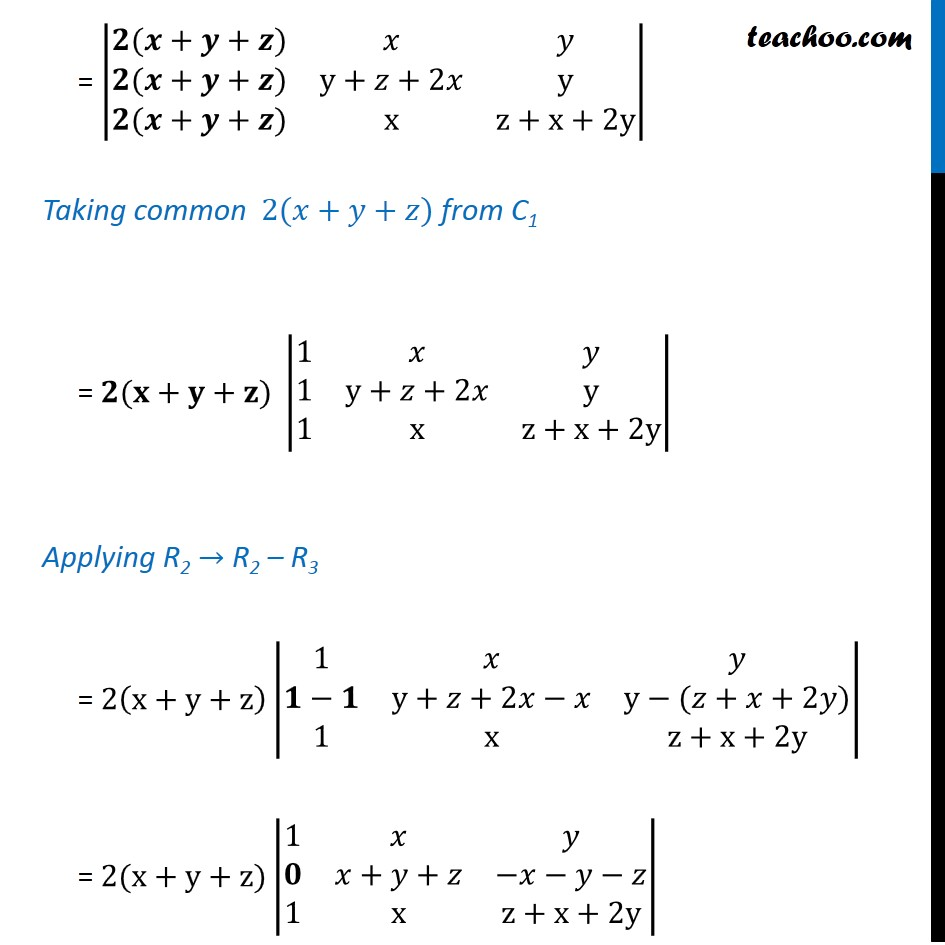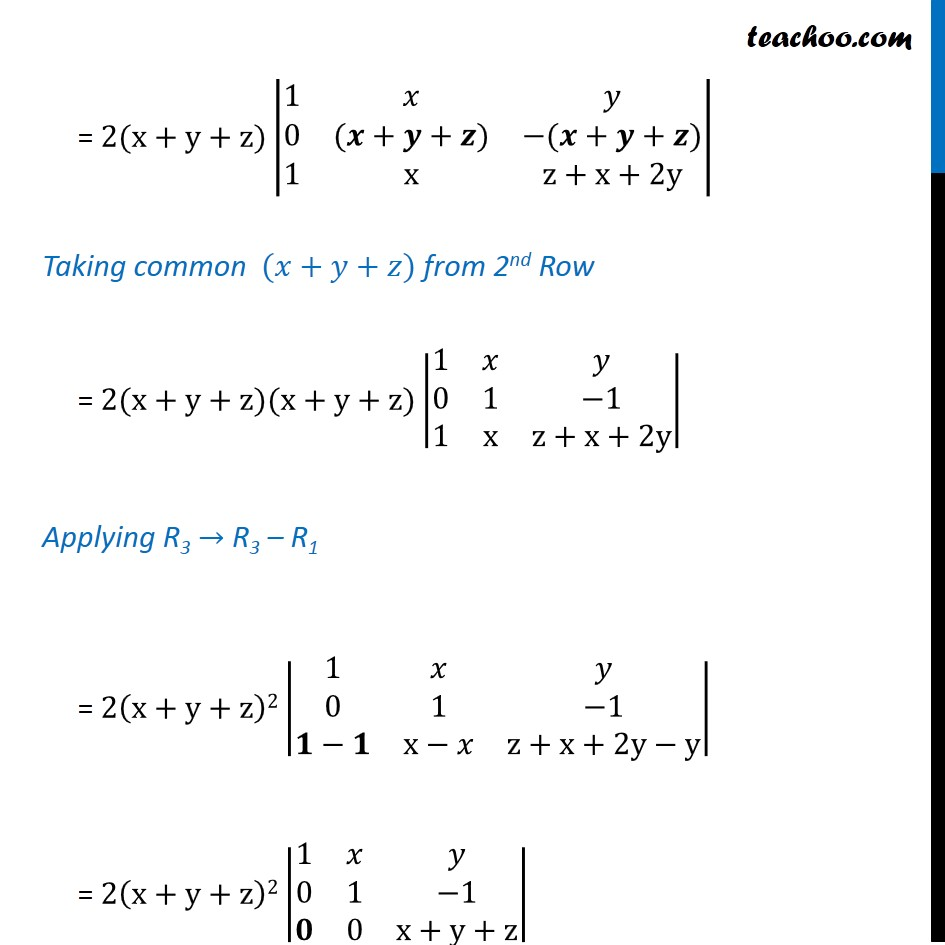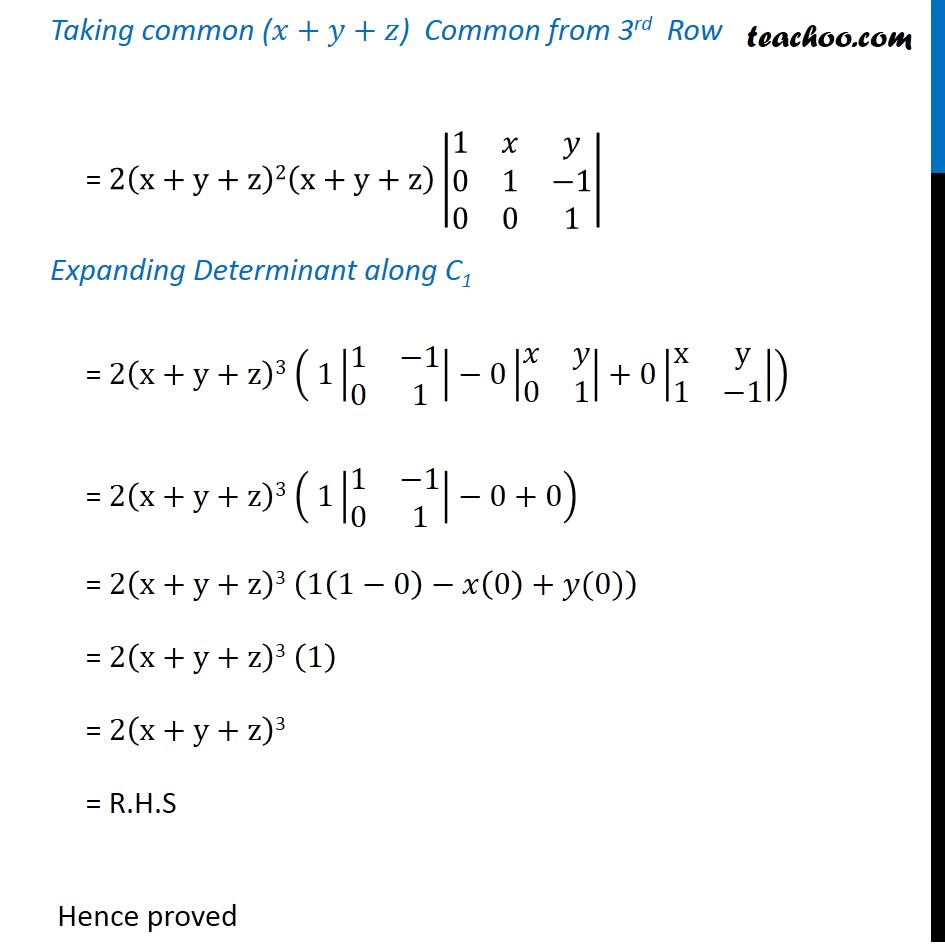Subscribe to our Youtube Channel - https://you.tube/teachoo

1. Chapter 4 Class 12 Determinants
2. Serial order wise
3. Ex 4.2

Transcript

Ex 4.2, 11 By using properties of determinants, show that: (i) |■8(a−b−c&2a&2a@2b&b−c−a&2b@2c&2c&c−a−b)| = (a + b + c)3 Solving L.H.S |■8(a−b−c&2a&2a@2b&b−c−a&2b@2c&2c&c−a−b)| Applying R1 → R1 + R2 + R3 = |■8(a−b−c+2𝑏+2𝑐&2a+b−c−a+2c&2a+2𝑏+𝑐−𝑎−𝑏@2b&b−c−a&2b@2c&2c&c−a−b)| = |■8(𝐚+𝐛+𝐜&𝐚+𝐛+𝐜&𝐚+𝒃+𝒄@2b&b−c−a&2b@2c&2c&c−a−b)| Taking common (a + b + c) from R1 = (a+b+c) |■8(1&1&1@2b&𝑏−𝑐−𝑎&2𝑏@2c&2𝑐&c−a−b)| Applying C1 → C1 – C2 = (a+b+c) |■8(𝟏−𝟏&1&1@2b−(b−c−a)&b−c−a&2𝑏@2c−2c&2𝑐&𝑐−𝑎−𝑏)| = (a+b+c) |■8(𝟎&1&1@b+c+a&b−c−a&2𝑏@0&2𝑐&𝑐−𝑎−𝑏)| Applying C2 → C2 – C3 = (𝑎+𝑏+𝑐)|■8(0&𝟏−𝟏&1@𝑏+𝑐+𝑎&𝑏−𝑐−𝑎−2𝑏&2𝑏@0&2𝑐−(𝑐−𝑎−𝑏)&𝑐−𝑎−𝑏)| = (𝑎+𝑏+𝑐)|■8(0&𝟎&1@𝑏+𝑐+𝑎&−𝑏−𝑐−𝑎&2𝑏@0&𝑎+𝑏+𝑐&𝑐−𝑎−𝑏)| Taking (a + b + c) common from C1 = (𝑎+𝑏+𝑐)(𝑎+𝑏+𝑐)|■8(0&0&1@1&−𝑏−𝑐−𝑎&2𝑏@0&(𝑎+𝑏+𝑐)&𝑐−𝑎−𝑏)| = (𝑎+𝑏+𝑐)2 |■8(0&0&1@1&−(𝒂+𝒃+𝒄)&2𝑏@0&(𝒂+𝒃+𝒄)&𝑐−𝑎−𝑏)| Taking common (a + b + c) from C2 = (𝑎+𝑏+𝑐)2(a+b+c) |■8(0&0&1@1&−1&2𝑏@0&1&𝑐−𝑎−𝑏)| Expanding determinant along R1 = (a + b + c)3 ( 0|■8(−1&2b@1&c−a−b)|−0|■8(1&2b@0&c−a−b)|+1|■8(1&−1@0&1)|) = (a + b + c)3 (0−0+1(1−0)) = (a + b + c)3 (1) = (a + b + c)3 Hence proved Ex 4.2, 11 By using properties of determinants, show that: (ii) |■8(x+y+2z&x&y@z&y+z+2x&y@z&x&z+x+2y)| = 2(x + y + z)3 Solving L.H.S |■8(x+y+2z&x&y@z&y+z+2x&y@z&x&z+x+2y)| Applying C1 → C1 + C2 + C3 = |■8(𝑥+𝑦+2𝑧+𝑥+𝑦&𝑥&𝑦@𝑧+𝑦+𝑧+2𝑥+𝑦&𝑦+𝑧+2𝑥&𝑦@𝑧+𝑥+𝑧+𝑥+2𝑦&𝑥&𝑧+𝑥+2𝑦)| = |■8(𝟐(𝒙+𝒚+𝒛)&𝑥&𝑦@𝟐(𝒙+𝒚+𝒛)&y+𝑧+2𝑥&y@𝟐(𝒙+𝒚+𝒛)&x&z+x+2y)| Taking common 2(𝑥+𝑦+𝑧) from C1 = 𝟐(𝐱+𝐲+𝐳) |■8(1&𝑥&𝑦@1&y+𝑧+2𝑥&y@1&x&z+x+2y)| Applying R2 → R2 – R3 = 2(x+y+z)|■8(1&𝑥&𝑦@𝟏−𝟏&y+𝑧+2𝑥−𝑥&y−(𝑧+𝑥+2𝑦)@1&x&z+x+2y)| = 2(x+y+z)|■8(1&𝑥&𝑦@𝟎&𝑥+𝑦+𝑧&−𝑥−𝑦−𝑧@1&x&z+x+2y)| = 2(x+y+z)|■8(1&𝑥&𝑦@0&(𝒙+𝒚+𝒛)&−(𝒙+𝒚+𝒛)@1&x&z+x+2y)| Taking common (𝑥+𝑦+𝑧) from 2nd Row = 2(x+y+z)(x+y+z)|■8(1&𝑥&𝑦@0&1&−1@1&x&z+x+2y)| Applying R3 → R3 – R1 = 2(x+y+z)2|■8(1&𝑥&𝑦@0&1&−1@𝟏−𝟏&x−𝑥&z+x+2y−y)| = 2(x+y+z)2|■8(1&𝑥&𝑦@0&1&−1@𝟎&0&x+y+z)| Taking common (𝑥+𝑦+𝑧) Common from 3rd Row = 2(x+y+z)2(x+y+z)|■8(1&𝑥&𝑦@0&1&−1@0&0&1)| Expanding Determinant along C1 = 2(x+y+z)3 ( 1|■8(1&−1@0&1)|−0|■8(𝑥&𝑦@0&1)|+0|■8(x&y@1&−1)|) = 2(x+y+z)3 ( 1|■8(1&−1@0&1)|−0+0) = 2(x+y+z)3 (1(1−0)−𝑥(0)+𝑦(0)) = 2(x+y+z)3 (1) = 2(x+y+z)3 = R.H.S Hence proved

Ex 4.2